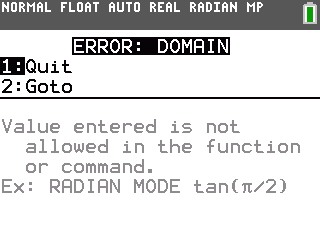# Knowledge Base

## Solution 34423: ERROR: DOMAIN When Attempting a Statistical Regression on the TI-84 Plus C Silver Edition.

### What does the ERROR: DOMAIN message mean when I perform a statistical regression on the TI-84 Plus C Silver Edition?

Statistical regressions include the following:

• LinReg() - Linear Regression
• CubicReg() - Cubic Regression
• QuartReg() - Quartic Regression
• LnReg() - Logarithmic Regression
• ExpReg() - Exponential Regression
• PwrReg() - Power Regression
• Logistic() - Logistic Regression
• SinReg() - Sinusoidal RegressionAn ERR:DOMAIN will be displayed when performing a statistical regression where the data used does not fit the model for that particular regression. The error indicates that either:

a) There is an answer and it is out of the range of the calculator.
b) There is no solution for the problem.

Please see the TI-84 Plus C Silver Edition guidebook for additional information.